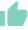# IRR VS ROIGaurav Seth
20 Likes | 391 days agoLikeShareAuthor is a Zfunds Verified ExpertGaurav Seth
Gurugram## IRR VS ROI

When it comes to computing the performance if the investments done, there are a very few metrics that are used more than the ROI (Return on Investment) and IRR (Internal Rate of Return)

## UNDERSTANDING

IRR is a metric that does not have any real formula in real life. It means that no predetermined formula can be used to determine IRR. The value that IRR seeks is the discount rate, which makes the NPV of the sum of inflows equal to the net cash invested initially. For instance, if we are going to get Rs 20,000 at the year end due to completion of a project, then the initial cash we should invest, keeping in mind that the discount rate is 15% is Rs 17,391.30 (Rs20,000/1.15).

The above instance makes it clear that IRR calculates the rate of discount, keeping in mind what the future NPV is going to be. The rate that makes the variance between current investment and the future NPV zero is the correct discount rate. It can be taken as an annualised return rate.

ROI is a metric that computes the percentage decrease or increase in return for a particular investment over a set time frame. It is also referred to as ROR (Rate of Return).  ROI can be computed using the formula : ROI = {(expected value - original cost)/original cost} X 100.

ROI can be computed for any type of activity when there is an investment and there is an outcome from the measurable investment. But ROI can be more accurate for a shorter tenure. If ROI has to be calculated for years to come, then it is quite difficult to accurately calculate a future outcome that is so far away.

ROI is much easier to calculate and hence is mostly used more than IRR. But the improvement in tech has made IRR. Computation to be done by the use of software. Hence IRR is also used more frequently nowadays.

## THE CRUX

Two of the most used techniques or metrics for the computation of the performance of the investments are IRR vs ROI. So, generally the metric that is going to be used for computation of investment returns depends on the additional costs that are required to be taken into consideration.

IRR vs ROI has its own set of weaknesses and strengths. So, many firms use both the ROI vs IRR to compute their budgets for capital needed. These two metrics are most significantly used in decision making when it comes to accepting a new project or rejecting her. This shows the significance or importance of these two metrics.